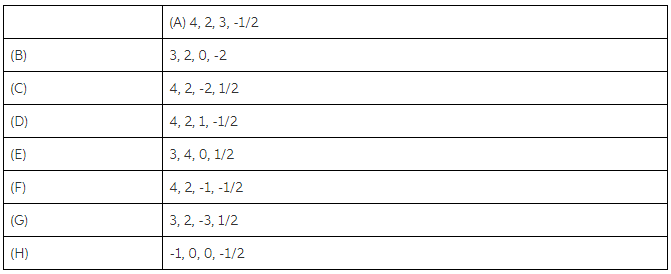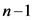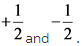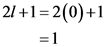# What is the only possible value of m? for an electron in an s orbital? Express your answer numerically

Part A
What is the only possible value of m? for an electron in an s orbital?

Part B
What are the possible values of m? for an electron in a d orbital?

Part C
Which of the following set of quantum numbers (ordered n, ?, m?, ms) are possible for an electron in an atom?

Check all that apply.Concepts and reason
The concept used to solve this problem is based on quantum numbers.
There are four set of quantum number namely, principal quantum number, n, azimuthal quantum number, l, magnetic quantum number, m and spin quantum number, s. Principal quantum number tells about the subshell in which electron is present. It values varies from 1 to n here, n is natural integer.
Azimuthal quantum number, l tells about the shape of orbital. Its values vary from 0 to, here, n is principal quantum number.

Fundamentals
For a correct set of quantum number, all 4 quantum numbers must satisfy their possible ranges. For example, azimuthal quantum number, l cannot be more than principal quantum number, n. Also, there are only two possible values of spin quantum number that isFor s orbital, value of azimuthal quantum number is zero. Therefore, magnetic quantum numberhas one value that is equal to zero.

Part A
Possible value offor an electron in s orbital is zero.

Total number of magnetic quantum numbers is calculated as 2l+1. Value of azimuthal quantum number is zero. Therefore, total number of magnetic quantum numbers will be:And it varies from -l to +l therefore, it is single value equals to zero.

Part B
Possible values offor an electron in d orbital are -2, -1, 0, 1 and 2.

Part C.1
The set of quantum numbers is not possible.

Part C.2
The set of quantum numbers is not possible.

Part C.3
The set of quantum numbers is possible.

Part C.4
The set of quantum numbers is possible.

Part C.5
The set of quantum numbers is not possible.

Part C.6
The set of quantum numbers is possible.

Part C.7
The set of quantum numbers is not possible.

Part C.8
The set of quantum numbers is not possible.